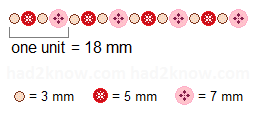# Necklace Bead Calculator

Bead 1 width = mm number in unit =
Bead 2 width = mm number in unit =
Bead 3 width = mm number in unit =
Bead 4 width = mm number in unit =
Bead 5 width = mm number in unit =
Bead 6 width = mm number in unit =

The length of a bead necklace varies according to the size of the beads and number used. If you make a necklace with beads of different sizes in a regular pattern, you can use simple arithmetic to compute how many beads or repeated pattern units it takes to reach a desired length.

Before you begin counting and measuring beads, you must first identify the basic unit of repetition in your necklace pattern. For instance, consider a necklace whose beads follow the pattern shown in the image below:In this sample, the basic unit is four beads long.

Once you have identified the pattern unit, you can measure its length. In the image above, the beaded necklace unit consists of one bead that is 7 mm long, one bead that is 5 mm long, and two beads that are 3 mm long. So the total length is 18 mm.

Next, use the length of the unit to determine how many units are required to reach a desired necklace length. For example, suppose you want a strand of beads that is 40 cm long, or about 15 ¾ inches long. To figure the number of units, divide the length of the necklace by the length of the pattern unit, keeping the same units of measurement. Since 40 cm is equivalent to 400 mm, you need

400/18 = 22.222 pattern units.

You can round the answer up or down to the nearest whole integer if you desire. For example, if you make a beaded necklace with 23 units that are 18 mm long, the total necklace length will be 414 mm, or 16 ¼ inches.